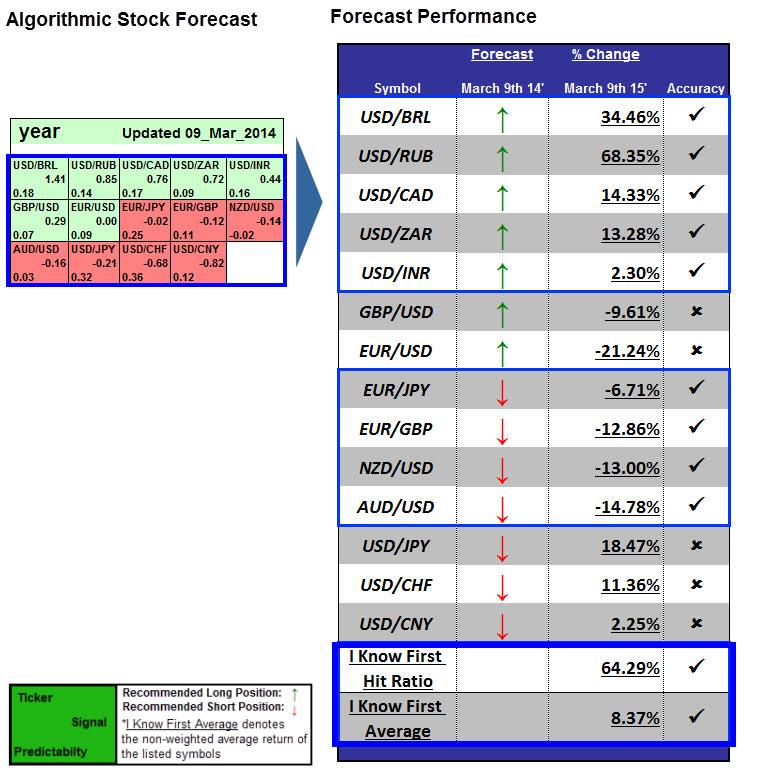## Currency Trends: 8.37% Average Return in 1 Year

### Currency Trends

The left-hand graph shows March 9th 2014 currency forecast which includes both long and short recommendations. The green boxes signify long signals and the red boxes signify short signals. The bright shades denote the strongest signals. The right-hand side shows the returns of the suggested currency pairs from March 9th 2014 to March 9th 2015.Forecast Length: 1 Year (12/09/14 – 03/09/15)## View More Currency Forecasts

How to interpret this diagram:

Algorithmic Currency Forecast: The table on the left is the currency forecast produced by I Know First’s algorithm. Each day, subscribers receive forecasts for six different time horizons. The currencies in the 1-month forecast may be different than those in the 1-year forecast. In the included table, only the relevant currencies have been included. A green box represents a positive forecast, while a red represents a negative forecast. The boxes are then arranged according to their respective signal and predictability values (see below for detailed definitions).

Forecast Performance:The table on the right compares the actual currency performance with I Know First’s prediction. The column titled “Forecast” shows which direction the algorithm predicted, and the column “% Change” shows the actual currency’s performance over the indicated time period. The “Accuracy” column shows a “√” if the algorithm correctly predicted the direction of the stock or an “x” if the forecast was incorrect. The “I Know First Hit Ratio” represents the algorithm’s accuracy when predicting the trend of the currency.

Signal: This indicator represents the predicted movement direction/trend; not a percentage or specific target price. The signal strength indicates how much the current price deviates from what the system considers an equilibrium or “fair” price.

Predictability: This value is obtained by calculating the correlation between the current prediction and the actual asset movement for each discrete time period. The algorithm then averages the results of all the prediction points, while giving more weight to recent performance. As the machine keeps learning, the values of P generally increase.